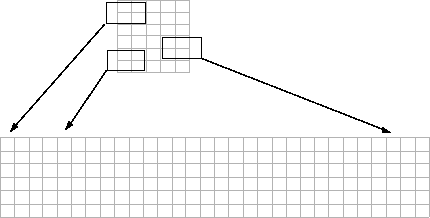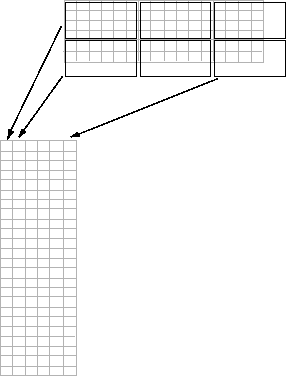## Use Column-wise Processing to Speed Up Sliding Neighborhood or Distinct Block Operations

Performing sliding neighborhood and distinct block operations column-wise, when possible, can reduce the execution time required to process an image.

For example, suppose the operation you are performing involves computing the mean of each block. This computation is much faster if you first rearrange the blocks into columns, because you can compute the mean of every column with a single call to the `mean` function, rather than calling `mean` for each block individually.

To use column processing, use the `colfilt` function. This function

1. Reshapes each sliding or distinct block of an image matrix into a column in a temporary matrix

2. Passes the temporary matrix to a function you specify

3. Rearranges the resulting matrix back into the original shape

### Using Column Processing with Sliding Neighborhood Operations

For a sliding neighborhood operation, `colfilt` creates a temporary matrix that has a separate column for each pixel in the original image. The column corresponding to a given pixel contains the values of that pixel's neighborhood from the original image.

The following figure illustrates this process. In this figure, a 6-by-5 image matrix is processed in 2-by-3 neighborhoods. `colfilt` creates one column for each pixel in the image, so there are a total of 30 columns in the temporary matrix. Each pixel's column contains the value of the pixels in its neighborhood, so there are six rows. `colfilt` zero-pads the input image as necessary. For example, the neighborhood of the upper left pixel in the figure has two zero-valued neighbors, due to zero padding.

colfilt Creates a Temporary Matrix for Sliding NeighborhoodThe temporary matrix is passed to a function, which must return a single value for each column. (Many MATLAB® functions work this way, for example, `mean`, `median`, `std`, `sum`, etc.) The resulting values are then assigned to the appropriate pixels in the output image.

`colfilt` can produce the same results as `nlfilter` with faster execution time; however, it might use more memory. The example below sets each output pixel to the maximum value in the input pixel's neighborhood, producing the same result as the `nlfilter` example shown in Implementing Linear and Nonlinear Filtering as Sliding Neighborhood Operations.

`I2 = colfilt(I,[3 3],"sliding",@max);`

### Using Column Processing with Distinct Block Operations

For a distinct block operation, `colfilt` creates a temporary matrix by rearranging each block in the image into a column. `colfilt` pads the original image with 0's, if necessary, before creating the temporary matrix.

The following figure illustrates this process. A 6-by-16 image matrix is processed in 4-by-6 blocks. `colfilt` first zero-pads the image to make the size 8-by-18 (six 4-by-6 blocks), and then rearranges the blocks into six columns of 24 elements each.

colfilt Creates a Temporary Matrix for Distinct Block OperationAfter rearranging the image into a temporary matrix, `colfilt` passes this matrix to the function. The function must return a matrix of the same size as the temporary matrix. If the block size is `m`-by-`n`, and the image is `mm`-by-`nn`, the size of the temporary matrix is `(m*n)`-by-`(ceil(mm/m)*ceil(nn/n))`. After the function processes the temporary matrix, the output is rearranged into the shape of the original image matrix.

This example sets all the pixels in each 8-by-8 block of an image to the mean pixel value for the block.

```I = im2double(imread("tire.tif")); f = @(x) ones(64,1)*mean(x); I2 = colfilt(I,[8 8],"distinct",f);```

The anonymous function in the example computes the mean of the block and then multiplies the result by a vector of ones, so that the output block is the same size as the input block. As a result, the output image is the same size as the input image.

#### Restrictions

You can use `colfilt` to implement many of the same distinct block operations that `blockproc` performs. However, `colfilt` has certain restrictions that `blockproc` does not:

• The output image must be the same size as the input image.

• The blocks cannot overlap.

For situations that do not satisfy these constraints, use `blockproc`.